# Module 1: INITIAL SETUP AND BASIC OPERATION

## Learning Objectives

After reading this lesson, you should be able to:

1. use the Command Window and understand its function,

2. control what appears in the Command Window,

3. access MATLAB documentation through the Command Window.

## What is the Command Window and how can I use it?

The Command Window (Figure 1) is the main window used to display the program output in MATLAB. We can also program directly into the Command Window; however, our code will not be saved in an m-file.Figure 1: The default MATLAB window configuration is shown with m-file open. The Command Window is at the bottom center.

Table 1: Commonly used mathematical operators.

Operation Syntax
Add +
Subtract -
Multiply *
Divide /
Power ^
Square Root sqrt()

This means you can quickly test a few lines of code, use it as a calculator, or check the value of a variable (see Figure 2). This also means it can be a useful tool when debugging (find errors in the code) in your program. To use basic mathematical operators and numerals, just input the statement and hit the enter key. Table 1 shows a few common mathematical operators commonly used in MATLAB. Note that when the answer is displayed, it is assigned the name, ans, by default.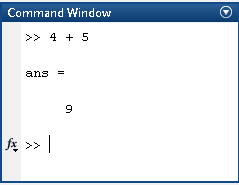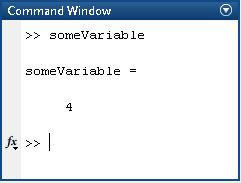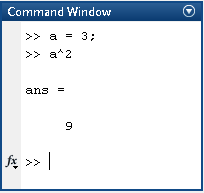Figure 2: Command Window used for a quick calculation (left), outputting the value of a previously defined variable (middle), and a quick and small program (right).

It is important to note that MATLAB does not understand what an equation is as known to you in a traditional sense. In MATLAB there is a variable name side and an expression side to any statement, and these sides are divided by an “equal to” sign. The variable name is always on the left side and the expression is always on the right side of an equal to sign. How this works is that MATLAB reads a variable name and attaches that variable name to what is on the right side of the equal to sign. This variable name is now associated with the number or expression on the right side of the equal to sign. If you do not assign the expression to a variable name, then MATLAB automatically assigns a default name, ans, to the expression.

## How can I suppress outputs in the Command Window?

The Command Window will display the outputs of all executable code that is in the m-file. In most cases, the programmer may only want the final solution to be displayed, suppressing outputs of all other lines. Suppressing variables can also decrease the run time of your program (if it is long). To make this possible, MATLAB has a special character that can be added to any line of the m-file – the suppression character. This suppression is made possible by inserting a semicolon (;) at the end of any line of code. It is important to note that although the output of a line followed by the semicolon (;) is not displayed in the Command Window, the calculation in the line is still being done “behind the scene” by MATLAB. An example of using this character to suppress outputs from the Command Window is shown in Example 1.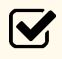Important Note: Suppressing a line of code will only change whether it outputs to the Command Window or not: it does not stop MATLAB from performing the operation.

### Example 1

Suppress variables from being output to the Command Window.

Solution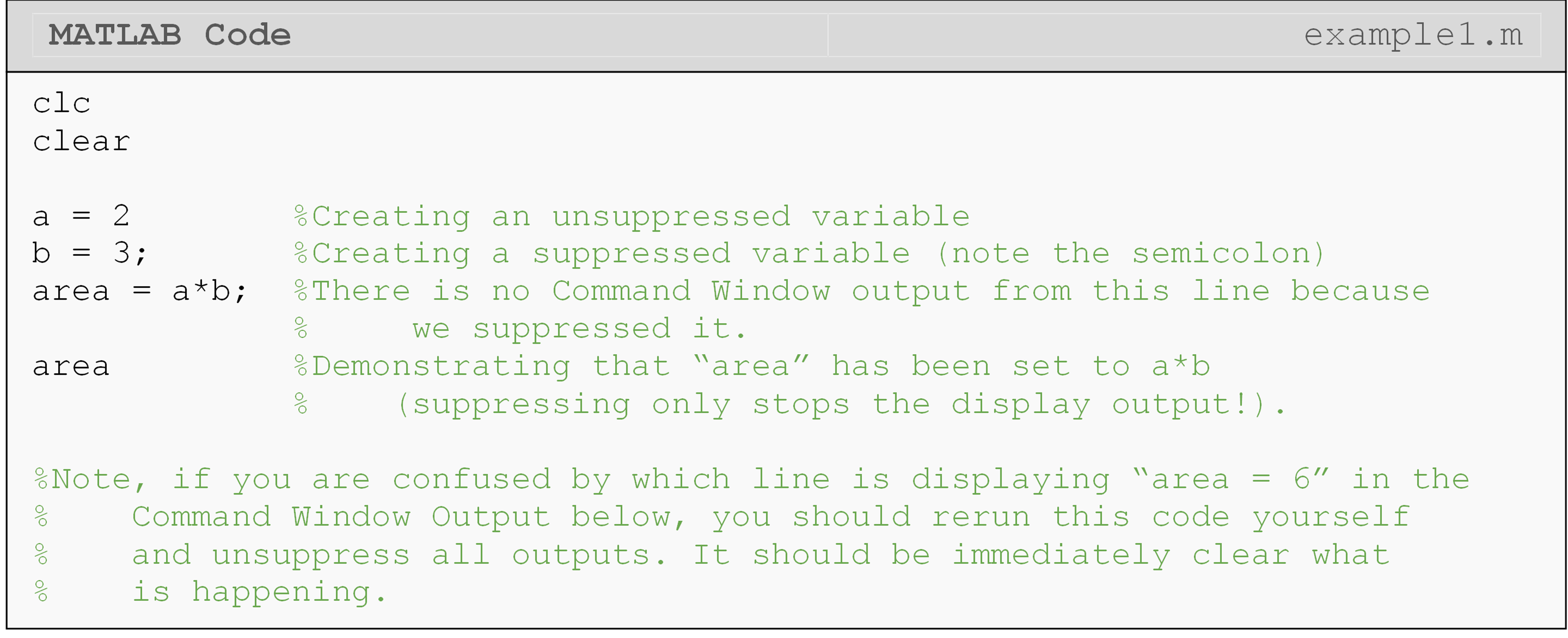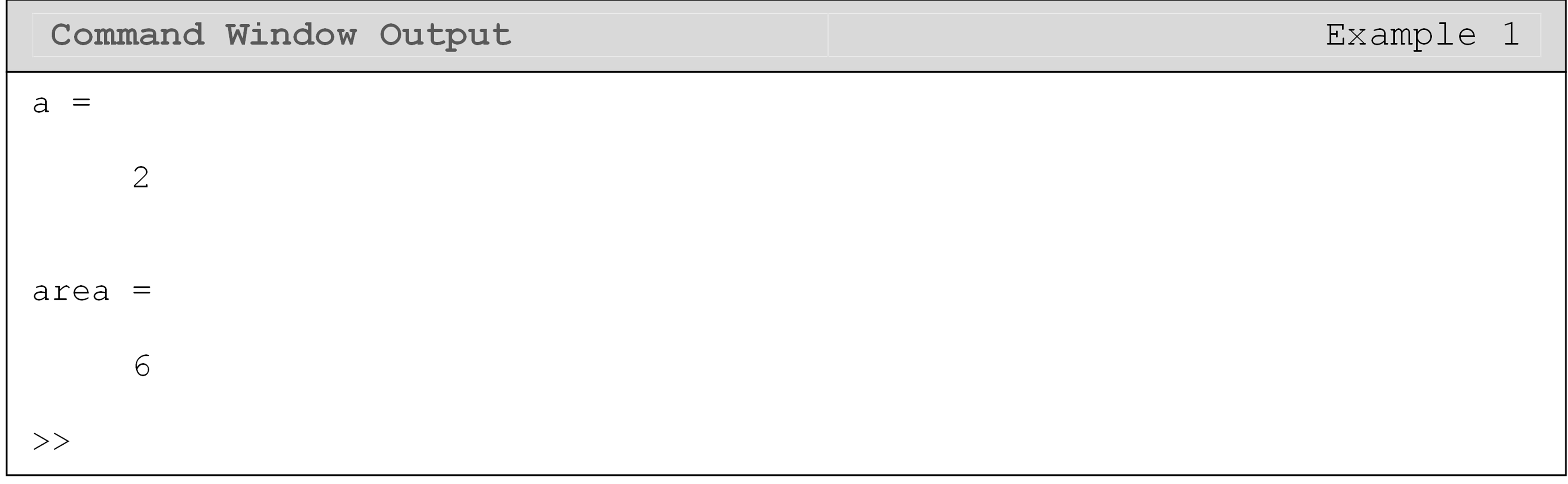## Can I view help from the Command Window?

If you cannot remember syntax/usage while you are programming, the help and doc commands can be used directly in the Command Window to pull up documentation on a specific MATLAB function or command.

The difference between help and doc is that help contains a summary of the documentation and displays directly in the Command Window (see Figure 3) while doc opens a new window with the full documentation page from MathWorks. Both are quick and easy ways to review documentation.

## Can the Command Window do it all?

The Command Window does have limitations. In fact, it is rarely used to perform mathematical operations or for doing MATLAB programming. The main reasons for this are that it is difficult to change expressions without overwriting them and displaying useful information in the Command Window is cumbersome. For the purposes of this book, the main role of the Command Window is to only display the output information from a MATLAB file (also called an m-file). Although it is important to understand the inner workings of the Command Window, the m-file is the basis of MATLAB programming as described in Lesson 1.5.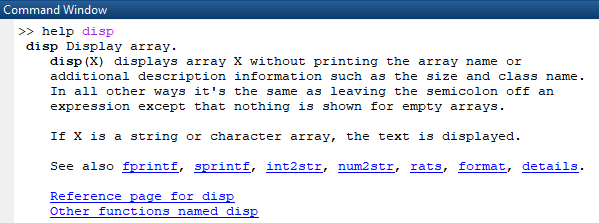Figure 3: Using the help command to retrieve documentation from the Command Window.

## Lesson Summary of New Syntax and Programming Tools

 Task Syntax Example Usage Suppress an output (Command still processed) ; code; Get summary of documentation for a MATLAB command or function help >>help disp Open documentation page for a MATLAB command or function doc >>doc disp Add two variables + a+b Subtract two variables - a-b Multiply two variables * a*b Divide two variables / a/b Raise a variable to a power ^ a^2 Take the square root of a variable sqrt() sqrt(a)

## Multiple Choice Quiz

(1). The MATLAB syntax to suppress output from the Command Window is

(a)  %

(b)  &

(c)  supp(' ')

(d)  ;

(2). Using the Command Window, 2^(4^2) will give the following output

(a)  16

(b)  256

(c)  512

(d)  65536

(3). Using the Command Window, 2^4^2 will give the following output

(a)  16

(b)  256

(c)  512

(d)  65536

(4). In the Command Window, if we enter:

>>d = 5;

>>a = d^2

then the output of the last line is

(a)  5

(b)  10

(c)  25

(d)  Undefined function or variable 'd'.

(5). In the Command Window, if we enter:

>>a = 5;

>>a = 6;

>>a

then the output of the last line will be:

(a)  5

(b)  5.5

(c)  6

(d)  11

## Problem Set

Use the Command Window to complete the following exercises.

(1). Find the output of $$b$$ given that $$a = 6$$ and $$b = 12a$$.

(2). Using Ohm’s law,

$V = i \times R$

find the electrical current $$i$$ passing through a resistor of resistance $$R=2 \times 10^{3}\ \Omega$$, and a voltage potential $$V=12\text{ V }( \text{DC})$$.

(3). Find the area ($$\text{in}^2$$) of a right-angled triangle that has a base measurement of 4 inches and an adjacent angle of $$32^{\circ}$$.

(4). Find the lift force in Newtons of an airfoil at a constant velocity (V) of 35 m/s, and in a fluid environment with a density ($$\rho$$) of $$1.247\ \text{kg/m}^3$$. The airfoil has an exposed area ($$A_{exp}$$) of $$2451\text{ cm}^{2}$$ and the coefficient of lift is found to be 0.81. For your solution, you may use the formula for the lift force as:

$\displaystyle F_{\text{Lift}} = \frac{1}{2}\rho A_{\text{exp}} C_{\text{lift}} V^{2}$

(5). Redo exercise 4 as follows. Find the lift force in Newtons using the same fluid density, exposed area, and lift coefficient as stated in Exercise 4, but choose the velocity first to be 25 m/s and then to be 50 m/s. Note that the values of fluid density, lift coefficient, and exposed area are already stored in MATLAB.